## If - Else Statement

If - Else statement is not an independent statement, you need to write it after an if statement.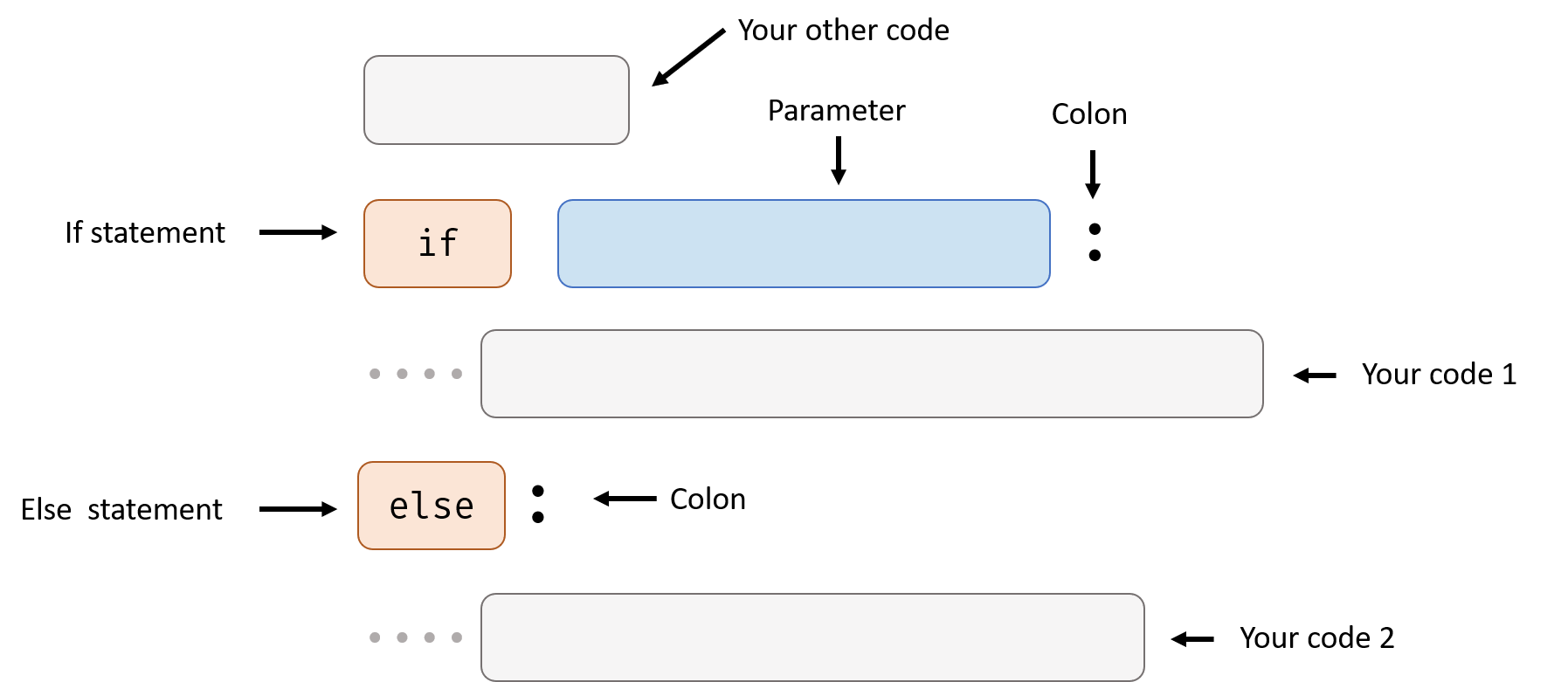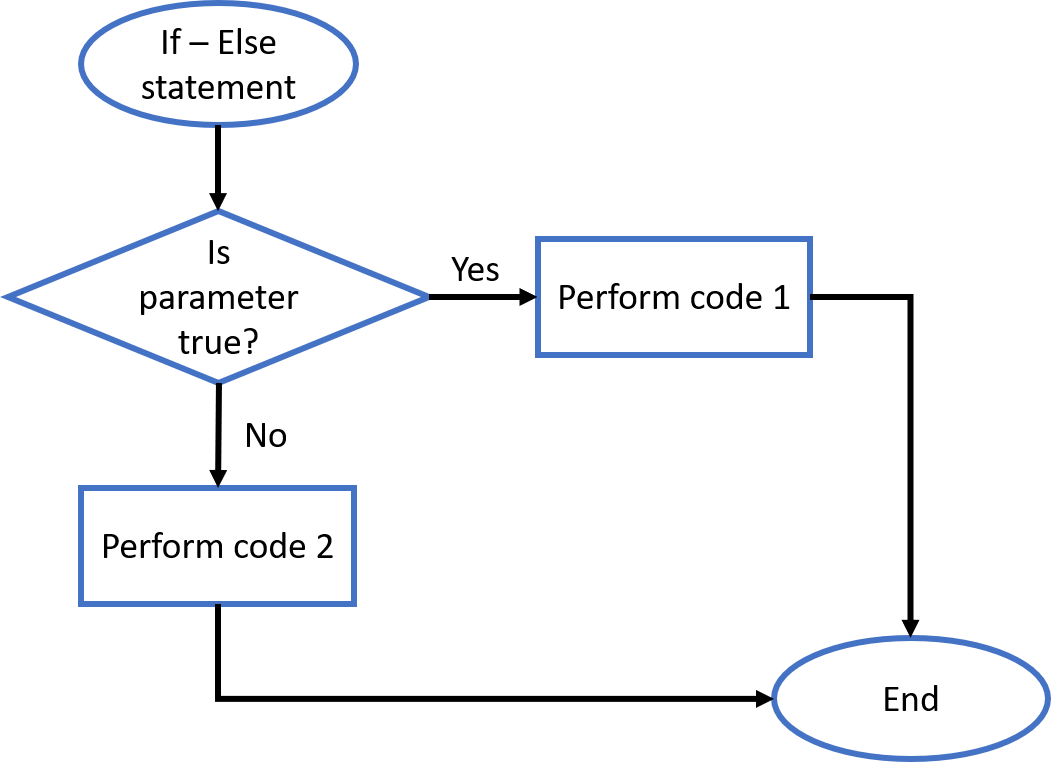When the parameter in the if statement is not True, python will execute the code under the else statement.

This is an example from Control Flow handout:

Now I want to let my program output a sentence if a is not greater than 5. I can do this:

Try changing a = 10 to a = 2, see what happens.

There can be only one else statement follow one is statement.

## If - Else If Statement

Else statement is useful, but sometimes you still want to check some condition before executing your second code. Else is statement is for this.

Else if statement is not an independent statement, you need to write it after an if statement.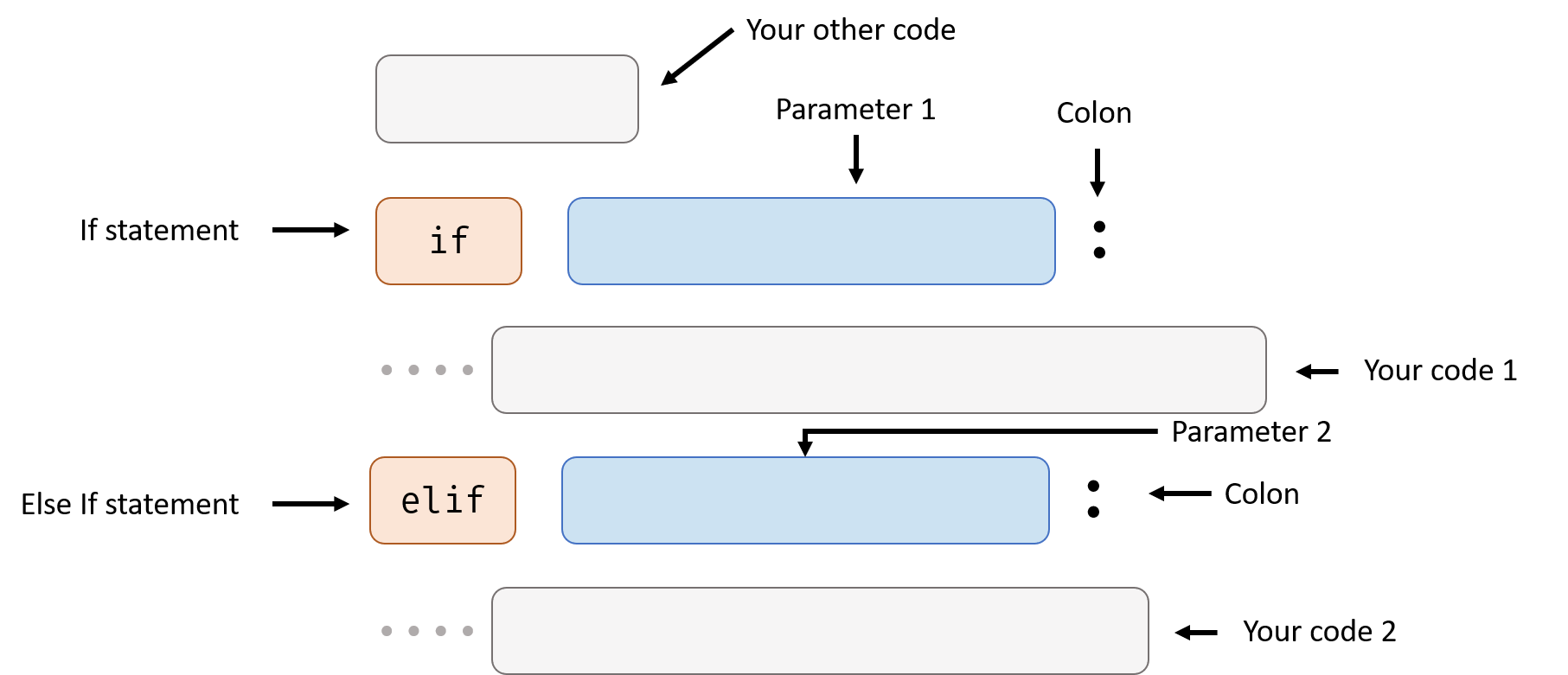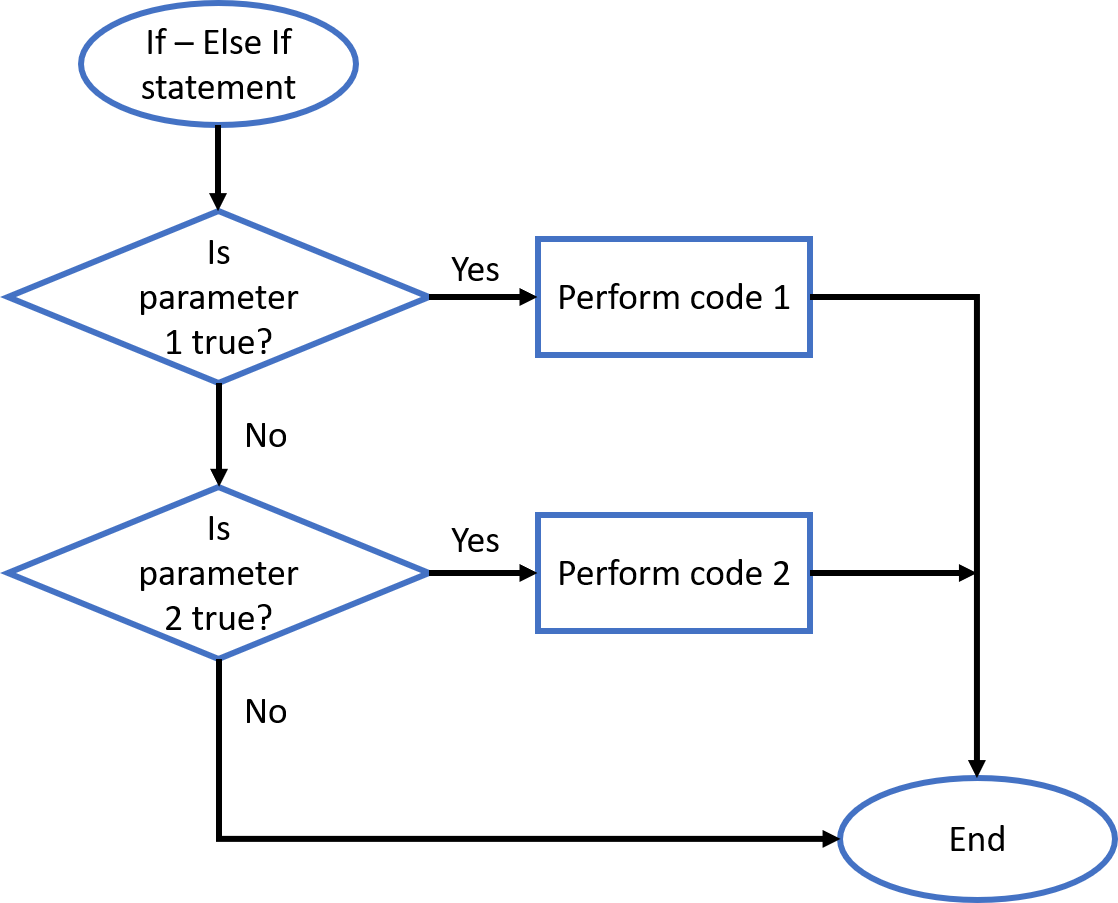This is an example from Control Flow handout:

Now, I know if variable a greater than 5. But in case it’s not, I want to know if it’s less than 3.

I can write like this: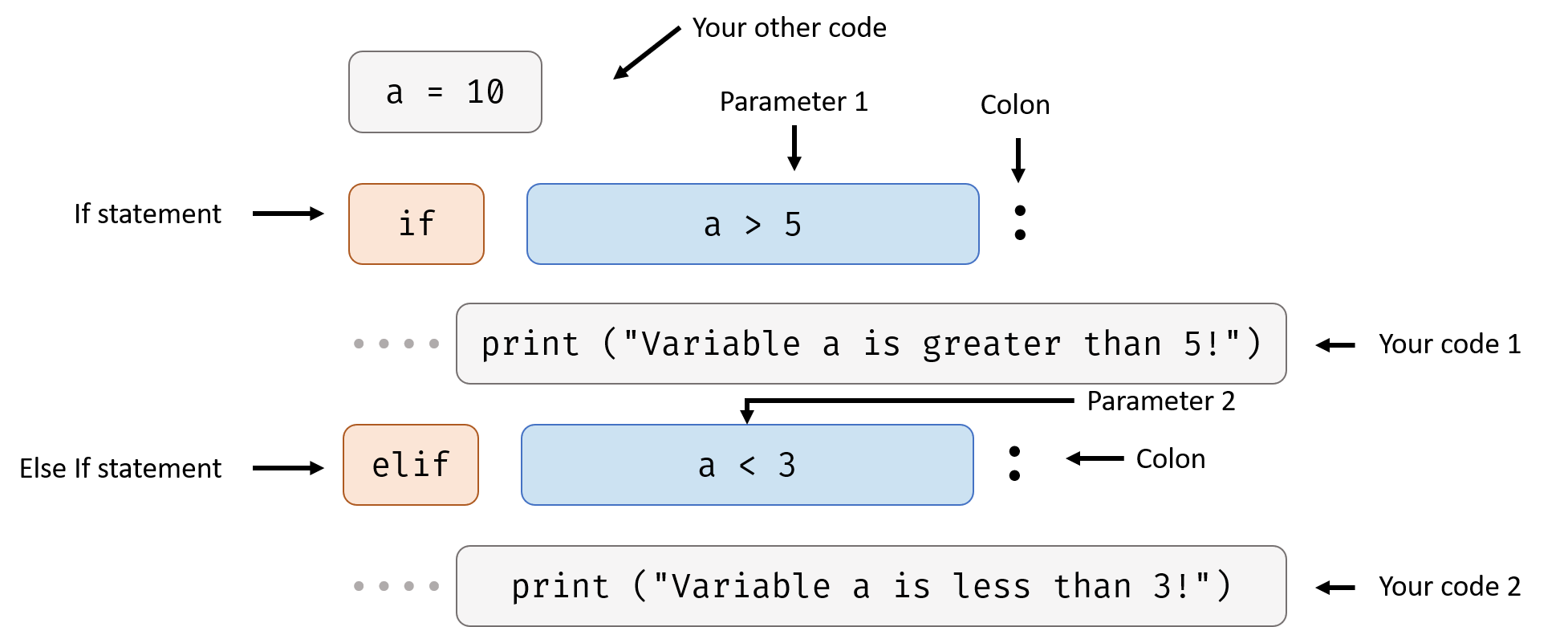Try to change a = 10 to a = 1, what will happen?

Maybe you are wondering what’s the difference between the example above this and the following example:

The difference is in the else if statement, if the first if parameter is true, python will not check the second parameter and skip running the second code at all. Here’s an example:

Try changing the elif to if or the number at the first line, see what happens.

You can use more than one else if statement under one if statement, For example:

## If - Else If - Else Statement

You can mix them together if you want.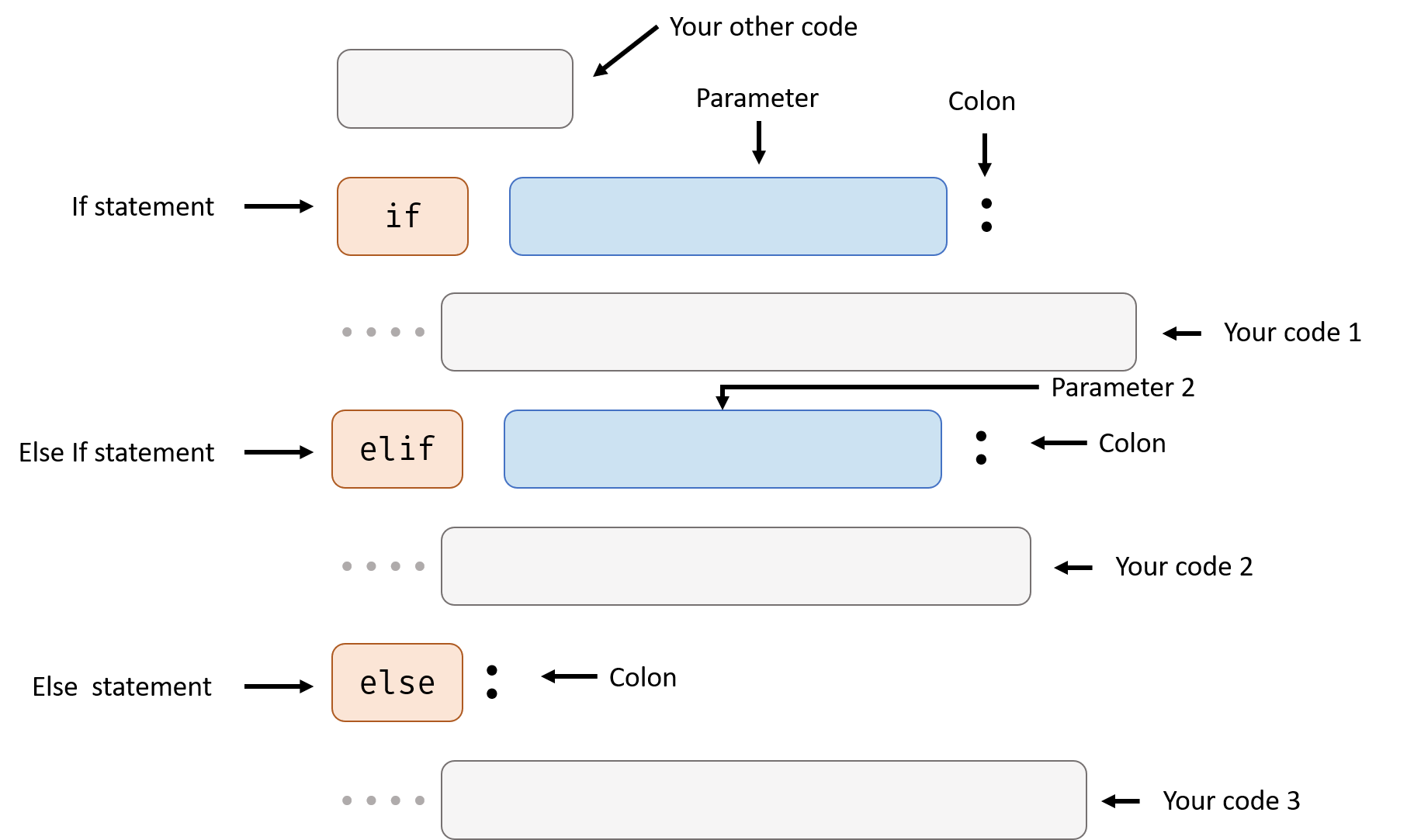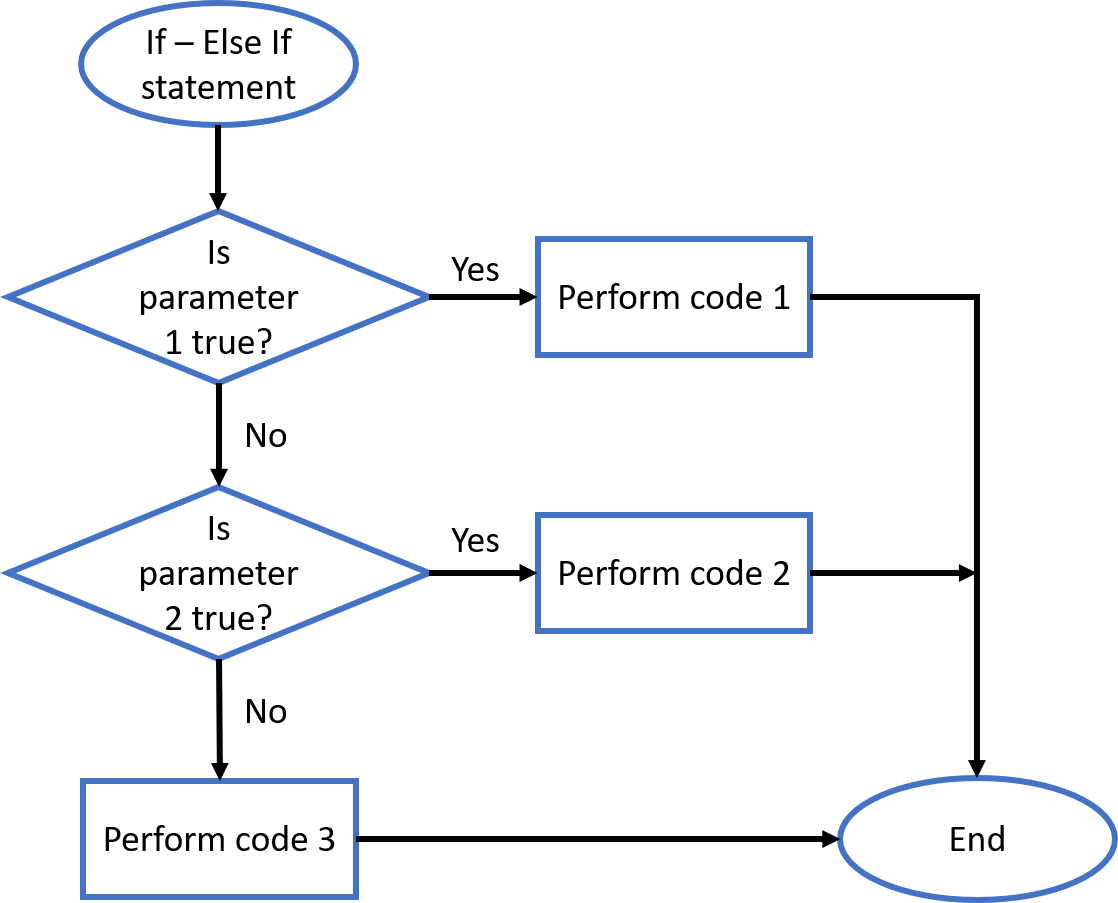Here’s some Example: